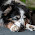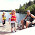## Wednesday, January 1, 2014

### No Exam - using Artifacts of Student Learning with an Interview.

In teaching MHF 4U advanced functions this semester, I cycled the curriculum which I have written about here . The students have written (will) 7 tests. Each test covered two or three strands.

There are also process expectations :

So instead of an exam I have asked my students to do the following:

In preparation for your final interview you are required to supply your teacher with 12 artifacts (one for each overall expectation-I combined two of them). Each one should be done on a separate page (one only).

The artifact should address the overall curriculum expectation and the process expectation(s) that is (are) tagged to it.

The artifact can be a homework question, an activity, an explanation, a diagram with comments, ……. really anything that would demonstrate your understanding of that curriculum expectation.

You should consider the following questions when choosing and presenting your artifacts:
• Why your artifact demonstrates the curriculum expectation? or Why did you choose it?
• In what ways does the artifact show what you have learned or understand? or In what ways does the artifact show your growth of learning?
• You chose the piece: What would you like me to look at or pay attention to?

All artifacts must be tagged (with stickies or highlights or markers or……) indicating evidence of the appropriate process expectations.

You should consider the following questions when tagging the evidence of the appropriate process expectations:
• Am I explaining why this tag demonstrates the process expectation? or Why did you choose it?
• In what ways does the tag show what you have learned or understand the process expectation? or In what ways does the tag show your growth of learning?
The Table looked like this:

 Curriculum Expectation Process Expectation(s) Strand: CHARACTERISTICS OF FUNCTIONS F1:Demonstrate an understanding of average and instantaneous rate of change, and determine, numerically and graphically, and interpret the average rate of change of a function over a given interval and the instantaneous rate of change of a function at a given point SELECTING TOOLS AND COMPUTATIONAL STRATEGIES Selects and uses tools and strategies to solve a problem F2:Determine functions that result from the addition, subtraction, multiplication, and division of two functions and from the composition of two functions, describe some properties of the resulting functions, and solve related problems CONNECTING Connects mathematical ideas to the context REPRESENTING Creates reasonable models to represent the problem (scale model, diagram, numbers, formulas, words) F3:Compare the characteristics of functions, and solve problems by modelling and reasoning with functions, including problems with solutions that are not accessible by standard algebraic techniques REPRESENTING Creates reasonable models to represent the problem (scale model, diagram, numbers, formulas, words) SELECTING TOOLS AND COMPUTATIONAL STRATEGIES Selects and uses tools and strategies to solve a problem `Strand: EXPONENTIAL AND LOGARITHMIC FUNCTIONS` EL1:Demonstrate an understanding of the relationship between exponential expressions and logarithmic expressions, evaluate logarithms, and apply the laws of logarithms to simplify expressions PROBLEM SOLVING / REASONING / PROVING Uses critical thinking to solve a problem REFLECTING Reflects on the processes used to solve the problem and reasonableness of answers EL2:Identify and describe some key features of the graphs of logarithmic functions, make connections among the numeric, graphical, and algebraic representations of logarithmic functions, and solve related problems graphically CONNECTING Connects mathematical ideas to the context REPRESENTING Creates reasonable models to represent the problem (scale model, diagram, numbers, formulas, words) EL3:Solve exponential and simple logarithmic equations in one variable algebraically, including those in problems arising from real-world applications CONNECTING Connects mathematical ideas to the context REFLECTING Reflects on the processes used to solve the problem and reasonableness of answers Strand: POLYNOMIAL AND RATIONAL FUNCTIONS PR1:Identify and describe some key features of polynomial functions, and make connections between the numeric, graphical, and algebraic representations of polynomial functions REPRESENTING Creates reasonable models to represent the problem (scale model, diagram, numbers, formulas, words) COMMUNICATING Expressing and organizing thinking Using mathematical vocabulary PR2:Identify and describe some key features of the graphs of rational functions, and represent rational functions graphically COMMUNICATING Expressing and organizing thinking Using mathematical vocabulary PR3:Solve problems involving polynomial and simple rational equations graphically and algebraically;  demonstrate an understanding of solving polynomial and simple rational inequalities CONNECTING Connects mathematical ideas to the context PROBLEM SOLVING / REASONING / PROVING Uses critical thinking to solve a problem Strand: TRIGONOMETRIC FUNCTIONS T1:Demonstrate an understanding of the meaning and application of radian measure COMMUNICATING Expressing and organizing thinking Using mathematical vocabulary T2:Make connections between trigonometric ratios and the graphical and algebraic representations of the corresponding trigonometric functions and between trigonometric functions and their reciprocals, and use these connections to solve problems CONNECTING Connects mathematical ideas to the context REPRESENTING Creates reasonable models to represent the problem (scale model, diagram, numbers, formulas, words) T3:Solve problems involving trigonometric equations and prove trigonometric identities SELECTING TOOLS AND COMPUTATIONAL STRATEGIES Selects and uses tools and strategies to solve a problem PROBLEM SOLVING / REASONING / PROVING Uses critical thinking to solve a problem

Ok MTBOS-here is what I am looking for.

What do you honestly think?

A special thanks to Sandra Herbst - whom I heard speak on Monday November 25th.
She inspired this!

1.Awesome as an exam! I did something similar but as a portfolio. But the idea was the same - a focus on the mathematical processes. Students were able to submit evidence throughout their learning to demonstrate that they have achieved the process expectations. There were some physical artifacts, but also some electronic documents as well (with pictures). I did it as an ongoing assessment, but I think the way that you're doing it as a substitute for exam is great!

What do the students think? When can they submit the artifacts?

Something that I did with the portfolio was that students could combine expectations if it's a problem that involved multiple ones. Maybe that's harder to do with the MHF4U expectations though?

Thanks for sharing!

2.Hey Jimmy, the students are submitting the artifacts on the last day of the course. They then tell me what strand(s) (two max) they want to focus on during the interview. Interviews take place on exam day 8-3. They can totally combine expectations. I am just bringing the artifacts to the interview as a starting point. Should be an interesting two days (as I have two sections). I'll let you know how it goes.

3.Alex, this looks awesome--I definitely want to try this sometime in the future.

A few questions:
(1) Have students practiced oral exams/interviews with you before? I could see this as a disadvantage for some students who might be intimidated by a teacher and who would do much better submitting something like a recording of themselves presenting the material or answering your questions (using something like Doceri).

(2) What about students copying each other's work and submitting that to you? How do you check for this when you might have a high number of submissions?

(3) How much time will you give (have you given) your students to complete this assignment?

If possible, I'd love to see examples of students' submissions after the assignment. I'd be scared to do this as a final just because the quality of work could vary and the open-endedness of it could be intimidating to students if you haven't done something like that before. However, if you provide them with examples, I find you often get 40 variations of your example instead of the wide-open creativity that'd be possible otherwise. Let us know how it goes!

1.Hi Jonathan,
Thanks for the interest.
1) This is my first crack at this. Students do a midterm interview with me but it is only about how things are going.
2) Copying could be an issue. Students will only interview on two strands max. It is about learning the expectations in the course. I intend to focus on the process expectations in the interview.
3) Students will be given 5 full periods to work on their "stuff". Students received the details about this on December 15th and the interview is the last week of January.

I will blog about the experience and include student samples. It should be interesting.